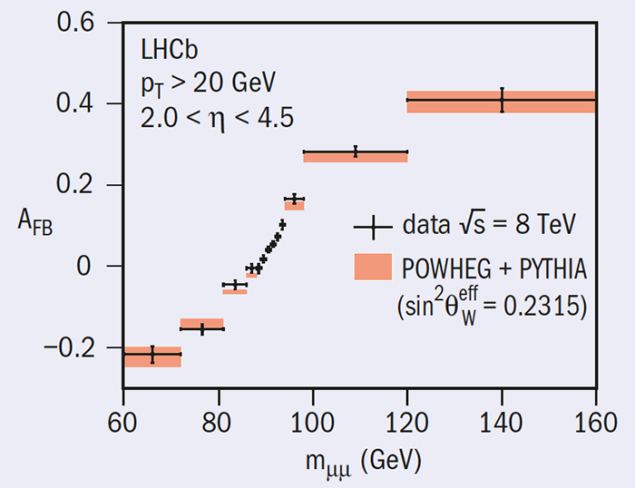# LHCb determines the electroweak mixing angle

28 October 2015

The electroweak mixing angle, θW, is a fundamental parameter of the Standard Model; it quantifies the relative strengths of electromagnetism and the weak force, and governs the Z-boson couplings to fermions. It is also something of a puzzle. The two most accurate determinations of the angle, carried out at LEP and SLD, are some three standard deviations different. More recent determinations at the Tevatron experiments, and by ATLAS and CMS at the LHC, have started to probe the difference. Now, LHCb has published a measurement based on LHC data taken in the forward region.Fig. 1. An example of the angular asymmetry, for data taken at 8 TeV centre-of-mass energies: measurement points are compared with Standard Model predictions (shaded).

LHCb has measured the asymmetry in the angular distribution of muons in dimuon final states, AFB, as a function of dimuon mass. The asymmetry depends on the squared sine function of the electroweak mixing angle, sin2θW, and can be used to determine a value for it once the directions of the interacting quark and antiquark, needed to define the sign of the asymmetry, are known. LHCb’s unique kinematic region benefits the analysis; dilution of the asymmetry is reduced as the incoming quark direction can be identified correctly 90% of the time, and theoretical uncertainties due to parton-density functions are lower than in the central region. In addition, LHCb’s ability to swap the direction of its magnetic field allows many valuable cross-checks to be performed.

An example of the angular asymmetry, for data taken at 8 TeV centre-of-mass energies, is shown in figure 1 as measurement points compared with a (shaded) Standard Model prediction. The effective electroweak mixing angle is found by comparing this asymmetry distribution with a series of Standard Model templates, corresponding to a range of values of angle, and choosing the one that best matches data. The analysis is performed on both the 7 and 8 TeV data sets, and the results are combined. The corresponding value of sin2θeffW is determined to be 0.23142±0.00073 (stat.)±0.00052 (sys.)±0.00056 (theory).

The value is one of the most precise measurements obtained at a hadron collider. Its accuracy is limited currently by statistics, and does not allow yet for a final word to be said on previous results from LEP, SLD, Tevatron and the LHC. In LHC Run 2 and beyond, there is scope to not just increase the number of events that can be analysed, but for improved parton-density functions (which dominate the theoretical error) to become available. The measurement should improve much further.